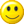# My Programs to Compute Fibonacci Numbers

The first program uses an efficient procedure that is based on repetition but takes longer to write. Programs based on repetition are called iterative.

The second program is shorter and easier to understand but it is rather inefficient. On my computer, it breaks for numbers higher than 29! Because it is a program that calls itself it is referred to as recursive.

To see my programs in action, please enter an integer number below and click on one of the buttons. On my computer, when I try the iterative version with a number greater than 1476 I get `Infinity` so, please, keep the numbers reasonably smallEnter a number:

 ```function iterativeFibonacci(n) { if (n == 0) return 0 if (n == 1) return 1 var term var small = 0 var large = 1 var i = 1 while (i < n) { term = large + small small = large large = term i = i + 1 } return term }``` ```function recursiveFibonacci(n) { if (n == 0) return 0 if (n == 1) return 1 return recursiveFibonacci(n - 1) + recursiveFibonacci(n - 2) }```

The following parabola is formed when we plot the Fibonacci numbers:The following curve is formed when we plot the approximation of the Golden Ratio obtained by dividing successive terms of the Fibonacci series: